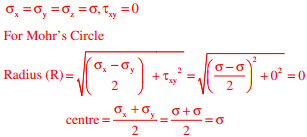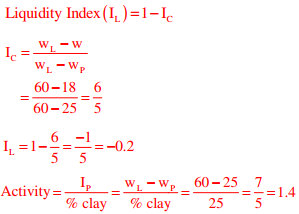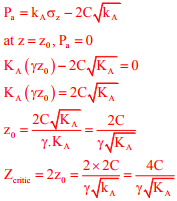# Geotechnical Engineering - Online Test

Q1. Group I lists the type of gain or loss of strength in soils. Group II lists the property or process responsible for the loss or gain of strength in soils.
Group I                                                                        Group II
P. Regain of strength with time                                   1. Boiling
R. Loss of strength due to upward seepage               3. Thixotropy
S. Loss of strength due to remolding                          4. Sensitivity
The correct match between Group I and Group II is

Explaination / Solution:
No Explaination.

Q2. A soil sample is subjected to a hydrostatic pressure, σ. The Mohr circle for any point in the soil sample would be
Explaination / Solution:Q3. The laboratory test on a soil sample yields the following results: natural moisture content = 18%, liquid limit = 60%, plastic limit = 25%, percentage of clay sized fraction = 25%. The liquidity index and activity (as per the expression proposed by skempton ) of the soil, respectively, are
Explaination / Solution:Q4. The old concert hall was demolished because of fears that the foundation would be affected by the construction tried to mitigate the impact of pressurized air pockets created by the excavation of large amounts of soil. But even with these safeguards, it was feared that the soil below the concert hall would not be stable. From this, one can infer that
Explaination / Solution:
No Explaination.

Q5. Bull's trench kiln is used in the manufacturing of
Explaination / Solution:
No Explaination.

Q6. The compound which is largely responsible for initial setting and early strength gain of Ordinary Portland Cement is
Explaination / Solution:
No Explaination.

Q7. In the consolidated undrained triaxial test on a saturated soil sample, the pore water pressure is zero
Explaination / Solution:
No Explaination.

Q8. A fine grained soil is found to be plastic in the water content range of 26-48%. As per Indian Standard Classification System, the soil is classified as
Explaination / Solution:

Soil is plastic in range of 26% to 48%. So, plastic limit =26%, liquid limit = 48% Since 35%
Q9. A vertical cut is to be made in a soil mass having cohesion c, angle of internal friction ϕ, and unit weight γ. Considering Ka and Kp as the coefficients of active and passive earth pressures, respectively, the maximum depth of unsupported excavation is
Explaination / Solution:Q10. The combined correction due to curvature and refraction (in m) for distance of 1 km on the surface of Earth is
Explaination / Solution:

C = 0.0673d2 = 0.0673×1